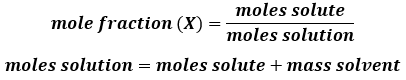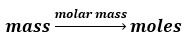# Problem: A solution is made containing 14.6 g of CH3OH in 185 g H2O.Calculate the mole fraction of CH3OH.

###### FREE Expert Solution

Recall the formula for mole fraction:Calculate the amount in moles of each compound first:

Solute = lesser amount = CH3OH

Solvent = greater amount = H2O97% (294 ratings)###### Problem Details

A solution is made containing 14.6 g of CH3OH in 185 g H2O.

Calculate the mole fraction of CH3OH.